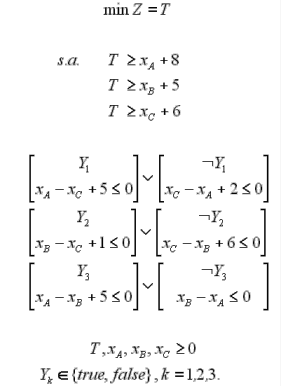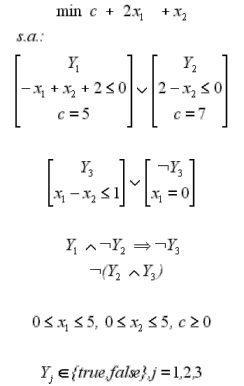# Examples

### Small Example 1This example corresponds to a Jobshop (Jobshop scheduling) problem, having three jobs (A,B,C) that must be executed sequentially in three steps (1,2,3), but not all jobs require all the stages, meaning that the jobs will be executed in a subset of stages. The processing time for each stage is given by the following table:

 Job/stage 1 2 3 A 5 - 3 B - 3 2 C 2 4 -

The objective is to obtain the sequence of task, which minimizes the completion time T. In order to obtain a feasible solution the clashes between the jobs must be eliminated. For more details about this formulation see Raman & Grossmann (1994).
LogMIP input file for this example

### Small Example 2Small example for illustration purposes. It is composed by two disjunctions each one with two terms.Each term of the first disjunction is handled by different variables. The first term is true if Y1 is true; the second term of the first disjunction is true if Y2 is true.The second disjunction is handled just for one variable: Y3. The first term applies if Y3 is true, the second if Y3 is false. The logic propositions indicates that:   1. If Y1 is true and Y2 false it implies that Y3 must be false.   2. Y2 and Y3 can not be both true at the same time. LogMIP input file for this example